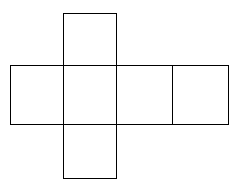## Project 2: Nets for Deltahedra

Definition 1: A net for a polyhedron is a diagonalized plane polygon which can be folded along the diagonals and assembled into a 3-dimensional polyhedron.

For example, shown below is one of the nets of the cube:There are, in fact, 10 other non-equivalent nets for the cube!

Definition 2: A deltahedron is a 3-dimensional polyhedron all of whose faces are equilateral triangles. (Many books reserve deltahedron for polyhedra which are convex.)

Problems

1. Find the different nets for various convex deltahedra. The easiest place to start is the tetrahedron. The next "smallest" example is the solid obtained by pasting two regular tetrahedra together along a face, and the next simplest after that is the octahedron. (How does the number of nets of the octahedron which are non-equivalent compare with the number of non-equivalent nets for the cube?)

2. Given a plane polygon P which can be triangulated into equilateral triangles. Can P serve as the net for two non-equivalent polyhedra all of whose faces are triangles? (See 2. in background section below.)

3. Given a plane polygon P which can be triangulated into equilateral triangles. Can P serve as the net for two non-equivalent convex deltahedra? Can the same triangulated polygon be folded into a convex and a non-convex deltahedron? Can the same triangulated polygon be folded into two different non-convex polyhedra?

4. Given a plane polygon P which can be triangulated into equilateral triangles.

Try to produce such a polygon P which can serve as the net of three non-equivalent polyhedra such that: two of the polyhedra are convex and the other is non-convex or two are non-convex and one is convex.

Background:

1. It is still an unsolved problem whether or not every convex 3-dimensional polyhedron has a net. The difficulty is that for some ways of trying to cut a polyhedron and open it up, the polygons will overlap when flattened into the plane.

2. Strictly speaking, a net requires pasting instructions as to which edges are to be pasted to each other, since otherwise there may be several different polyhedra that can be assembled with the same net. This fact is explicitly pointed out in the paper "Polyhedra with convex nets" by G. C. Shephard.

3. Shephard in the paper above raises the question of which polyhedra have a combinatorially equivalent polyhedron with a net whose shape is a convex polygon. He gets a variety of results concerning this question.

Extensions:

1. Investigate other problems which involve nets for polyhedra. You might consider the case where all the faces of the polyhedron are rhombuses. (Example: Find all the inequivalent nets for a solid combinatorially equivalent to a cube but whose faces are all rhombuses).

References:

1. Beck, A., and M. Bleicher, and D. Crowe, Excursions into Mathematics, Worth, New York.

2. Pearce, P. and S. Pearce, Polyhedra Primer, Van Nostrand Reinhold, New York, 1978.

3. Shephard, G., Convex polytopes with convex nets, Math. Proceedings Cambridge Philosophical Soc., 78 (1975) 389-403.

Joseph Malkevitch
Department of Mathematics and Computing
York College (CUNY)
Jamaica, New York 11451-0001
email: malkevitch@york.cuny.edu
(Comments and results related to the project above are welcome.)

Acknowledgements
Some of this work was prepared with partial support from the National Science Foundation (Grant Number: DUE 9555401) to the Long Island Consortium for Interconnected Learning (administered by SUNY at Stony Brook, Alan Tucker, Project Director).